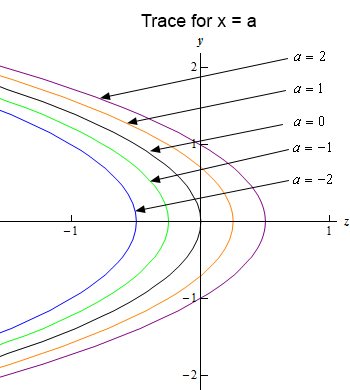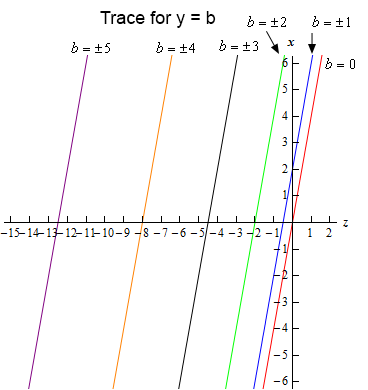Paul's Online Notes
Home / Calculus III / 3-Dimensional Space / Functions of Several Variables
Show Mobile Notice Show All Notes Hide All Notes
Mobile Notice
You appear to be on a device with a "narrow" screen width (i.e. you are probably on a mobile phone). Due to the nature of the mathematics on this site it is best views in landscape mode. If your device is not in landscape mode many of the equations will run off the side of your device (should be able to scroll to see them) and some of the menu items will be cut off due to the narrow screen width.

### Section 12.5 : Functions of Several Variables

9. Identify and sketch the traces for the following function.

$4z + 2{y^2} - x = 0$

Show All Steps Hide All Steps

Start Solution

We have two traces. One we get by plugging $$x = a$$ into the equation and the other we get by plugging $$y = b$$ into the equation. Here is what we get for each of these.

\begin{align*}x = a: & \hspace{0.25in}4z + 2{y^2} - a = 0 & \to \hspace{0.25in} & z = - \frac{1}{2}{y^2} + \frac{a}{4}\\ y = b: & \hspace{0.25in}4z + 2{b^2} - x = 0 & \to \hspace{0.25in} & x = 4z + 2{b^2}\end{align*} Show Step 2

Okay, we’re now into a realm that many students have issues with initially. We no longer have equations in terms of $$x$$ and $$y$$. Instead we have one equation in terms of $$x$$ and $$z$$ and another in terms of $$y$$ and $$z$$.

Do not get excited about this! They work the same way that equations in terms of $$x$$ and $$y$$ work! The only difference is that we need to make a decision on which variable will be the horizontal axis variable and which variable will be the vertical axis variable.

Just because we have an $$x$$ doesn’t mean that it must be the horizontal axis and just because we have a $$y$$ doesn’t mean that it must be the vertical axis! We set up the axis variables in a way that will be convenient for us.

In this case since both equation have a $$z$$ in them we’ll let $$z$$ be the horizontal axis variable for both of the equations.

So, given that convention for the axis variables this means that for the $$x = a$$ trace we’ll have a parabola that opens to the left with vertex at $$\left( {\frac{a}{4},0} \right)$$ and for the $$y = b$$ trace we’ll have a line with slope of 4 and an $$x$$-intercept at $$\left( {0,2{b^2}} \right)$$.

Show Step 3

Below is a sketch for each of the traces.